﻿ Simulative Analysis of Emitted Carbon during Gas Flaring Based on Quantified Magnitudes of Produced and Flared Gases

### Simulative Analysis of Emitted Carbon during Gas Flaring Based on Quantified Magnitudes of Produced ...

C. I. Nwoye, I. E. Nwosu, N. I. Amalu, S. O. Nwakpa, M. A. Allen, W. C. OnyiaOPEN ACCESSPEER-REVIEWED

## Simulative Analysis of Emitted Carbon during Gas Flaring Based on Quantified Magnitudes of Produced and Flared Gases

C. I. Nwoye1,, I. E. Nwosu2, N. I. Amalu3, S. O. Nwakpa1, M. A. Allen4, W. C. Onyia5

1Department of Metallurgical and Materials Engineering, Nnamdi Azikiwe University Awka, Anambra State, Nigeria

2Department of Environmental Technology, Federal University of Technology, Owerri, Nigeria

3Project Development Institute Enugu, Nigeria

4Department of Mechanical Engineering, Micheal Okpara University, Umuahia, Abia State, Nigeria

5Department of Metallurgical and Materials Engineering, Enugu State University of Science &Technology, Enugu, Enugu State, Nigeria

### Abstract

This paper presents a simulative analysis of emitted carbon during gas flaring based on quantified magnitudes of produced and flared gases. Results from both experiment and model prediction show that the quantity of emitted gas increases with increase in both total gas produced (TGP) and total gas flared (TGF). A two-factorial model was derived, validated and used for the empirical analysis. The derived model showed that emitted carbon is a linear function of TGP and TGF. The validity of the derived model expressed as: ξ = 0.0513 ₰ + 0.0776 ϑ + 30.7738 was rooted in the model core expression ξ - 30.7738 = 0.0513 ₰ + 0.0776 ϑ where both sides of the expression are correspondingly approximately equal. Results from evaluations indicated that the standard error incurred in predicting emitted carbon for each value of the TGP & TGF considered, as obtained from experiment, derived model and regression model were 14.2963, 7.4141 and 14.823 & 1.3657, 7.4084 and 0.0039 % respectively. Further evaluation indicates that emitted carbon per unit TGF as obtained from experiment; derived model and regression model were 0.155, 0.154 and 0.155 Tonnes/Mscfd-1 respectively. Comparative analysis of the correlations between emitted carbon and TGP & TGF as obtained from experiment; derived model and regression model indicated that they were all > 0.99. The maximum deviation of the model-predicted emitted carbon (from experimental results) was less than 3%. This translated into over 97% operational confidence for the derived model as well as over 0.97 reliability response coefficients of emitted carbon to TGP and TGF.

### At a glance: Figures

1234
Prev Next

• Nwoye, C. I., et al. "Simulative Analysis of Emitted Carbon during Gas Flaring Based on Quantified Magnitudes of Produced and Flared Gases." American Journal of Mining and Metallurgy 3.1 (2015): 9-14.
• Nwoye, C. I. , Nwosu, I. E. , Amalu, N. I. , Nwakpa, S. O. , Allen, M. A. , & Onyia, W. C. (2015). Simulative Analysis of Emitted Carbon during Gas Flaring Based on Quantified Magnitudes of Produced and Flared Gases. American Journal of Mining and Metallurgy, 3(1), 9-14.
• Nwoye, C. I., I. E. Nwosu, N. I. Amalu, S. O. Nwakpa, M. A. Allen, and W. C. Onyia. "Simulative Analysis of Emitted Carbon during Gas Flaring Based on Quantified Magnitudes of Produced and Flared Gases." American Journal of Mining and Metallurgy 3, no. 1 (2015): 9-14.

 Import into BibTeX Import into EndNote Import into RefMan Import into RefWorks

### 1. Introduction

The inherent negative impact of air pollution on the environment and ecosystem has raised the need to understudy the various ways in which air could be polluted, the air pollutants as well as possible control measures.

These air pollutants could be primary or secondary. The primary pollutants are emitted directly from processes ie carbon monoxide gas from motor vehicle exhaust or sulphur dioxide released from factories as well as ash from a volcanic eruption. Secondary pollutants are not emitted directly. Rather, they form in the air when primary pollutants react or interact. An important example of a secondary pollutant is ground level ozone - one of the many secondary pollutants that make up photochemical smog.

Gas flaring is the burning of natural gas and other petroleum in flare stacks of upstream oil companies in oil fields during operations. Research  has shown that gas flaring is the singular and most common source of global warming; contributing to emissions of carbon monoxide, nitrogen (ii) oxide and methane gas.

Reports [2, 3, 4] have shown that the presence of pollutants in the atmosphere causes adverse effects on human health and damages to structures. Similar studies  have also shown that incomplete combustion do not only contribute to global warming and climate change, as green house gases (GHG), but have major adverse health impacts including acute respiratory infections, chronic obstructive pulmonary disease, asthma, nasopharyngeal and laryngeal cancer.

Studies [2, 3] have revealed that primary pollutants undergo chemical reactions to produce a wide variety of secondary pollutants when they are exposed to sunshine, production of Ozone (O3) being most typical. The researchers further typified ozone as an indicator of air quality in urban atmospheres besides the health problems its molecules could cause.

Gas flaring also affects the soil pH. This results to heterogeneous levels of acidity and alkalinity within the area of the flaring process. The low soil pH values at the flare points is suspected to have been as a result of the presence of flare which produced acidic oxides of carbon and nitrogen. These oxides invariably forms carbonic and nitric acids on dissolving in rain water in accordance with research with past findings [6, 7].

It has been shown  that emission of a variety of compounds such as volatile organic compounds (VOC’s), polycyclic aromatic hydrocarbon (PAH’s) as well as sooth occur when the flaring process ensues with incomplete combustion.

Following investigation  on the Alberta Research Council (ARC) report regarding the compounds predominantly present during incomplete combustion of flared gases, it was discovered that Volatile Organic Compounds (VOC’s) and Polycyclic Aromatic Hydrocarbon (PAH’s) are the predominant compounds.

It is disheartening that since 1988, neither the Federal Environmental Protection Agency (FEPA) nor the Department of Petroleum Resources (DPR) has implemented anti-flaring policies for excess associated gases nor have they monitored the emissions to ensure compliance with standards .

The challenges associated with the control of gas flaring has been specifically reported [8, 9] to involve achievement of zero-carbon emissions (through application of (CCS)) since carbon is the primary constituent of the flared gas in the form VOC’s, PAH’s and sooth. These reports indicate that there are no difficulties encountered in removing the other components. There is a great challenge over human health and global warming risk posed by small level leakages over long periods during storage of captured CO2, as well as transportation of the CO2 without any form of leakage to locations were it is needed for other operations. The high cost of this technology is the major factor militating against global conformity to zero-carbon emissions since developing countries cannot withstand the financial implication of the project. The adaptability of the technology to easily maintainable conditions should also be considered. This is because the capture technology is difficult to maintain though it is economically feasible under specific conditions. Based on the foregoing, collaborative efforts are required between chemists and chemical engineers to overcome these challenges by considering a cheaper way of achieving CCS technology, a better mode of storing and transporting captured CO2.

Successful attempts [11, 12, 13] have been made to remove some these gases during flaring to ensure cleaner atmosphere at flaring sites.

The present work aims at carrying out a simulative analysis of emitted carbon during gas flaring based on quantified magnitudes of gas produced and flared

### 2. Materials and Method

A comprehensive survey of selected communities affected by gas flaring was carried out and their environments carefully monitored for observation. In these communities selected gas locations were considered for the study. Details of the experimental procedures are as stated in past report .

### 3. Results and Discussions

Table 1 indicates that the quantity of emitted carbon increases with increase in both total produced and total flared gases.

#### Table 1. Variation of emitted carbon with total flared gas and total gas produced 

3.1. Model Formulation

Computational analysis (using C-NIKBRAN: ) of results in Table 1 indicates that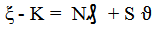(1)

Substituting the values of K, N and S into equation (1) reduces it to;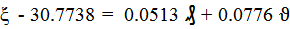(2)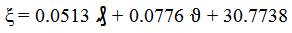(3)

Where

K, N and S, are equalizing constants

(ξ) = Carbon emitted (Tonnes)

(₰) = Total gas produced (Mscf/d)

(ϑ) = Total flared gas (Mscf/d)

3.1.1. Boundary and Initial Conditions

The ranges of carbon emitted, total gas produced and flared are 164.61-990.3 tonnes, 1062-9009.35 and 1062-6389.02 (Mscf/d) respectively. The flow rate of the gas was assumed constant.

3.1.2. Model Validation

#### Table 2. Variation of ξ - 30.7738 with 0.0513 ₰ + 0.0776 ϑ

The validity of the derived model was rooted in equation (2) where both sides of the equation are correspondingly approximately almost equal. Furthermore, equation (2) agrees with Table 2 following the values of ξ - 30.7738 and 0.0513 ₰ + 0.0776 ϑ evaluated from Table 1.

Furthermore, the derived model was validated by comparing the model-predicted emitted carbon and that obtained from the experiment. This was done using the 4th Degree Model Validity Test Techniques (4th DMVTT); statistical graphical, computational and deviational analysis.

Figure 1. Variation of emitted carbon with total gas produced as obtained from experiment 
3.2. Statistical Analysis
3.2.1. Standard Error (STEYX)

The standard errors incurred in predicting emitted carbon for each value of the TGP & TGF considered as obtained from experiment and derived model were 14.2963 and 7.4141 & 1.3657 and 7.4084 % respectively. The standard error was evaluated using Microsoft Excel version 2003.

3.2.2. Correlation (CORREL)

The correlation coefficient between emitted carbon and TGP & TGF were evaluated (using Microsoft Excel Version 2003) from results of the experiment and derived model. These evaluations were based on the coefficients of determination R2 shown in Figure 1 - Figure 4.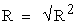(4)

The evaluated correlations are shown in Table 3 and Table 4. These evaluated results indicate that the derived model predictions are significantly reliable and hence valid considering its proximate agreement with results from actual experiment.

#### Table 3. Comparison of the correlations evaluated from derived model predicted and experimental results based on TGP

Comparative analysis of the correlations between emitted carbon and TGP & TGF as obtained from experiment; derived model and regression model indicated that they were all > 0.99.

#### Table 4. Comparison of the correlations evaluated from derived model predicted and experimental results based on TGF

3.3. Graphical Analysis

Comparative graphical analysis of Figure 5 and Figure 6 show very close alignment of the curves from the experimental (ExD) and model-predicted (MoD) emitted carbon.

It is strongly believed that the degree of alignment of these curves is indicative of the proximate agreement between both experimental and model-predicted values of the emitted carbon.

3.3.1. Comparison of Derived Model with Standard Model

The validity of the derived model was further verified through application of the Least Square Method (LSM) in predicting the trend of the experimental results. Comparative analysis of Figure 7 and Figure 8 shows very close alignment of curves and areas covered by emitted carbon, which precisely translated into significantly similar trend of data point’s distribution for experimental (ExD), derived model (MoD) and regression model-predicted (ReG) results of emitted carbon.

Also, the calculated correlations (from Figure 7 and Figure 8) between emitted carbon and TGP & TGF for results obtained from regression model gave 0.9992 & 1.0000 respectively. These values are in proximate agreement with both experimental and derived model-predicted results. The standard errors incurred in predicting emitted carbon for each value of the TGP & TGF considered as obtained from regression model were 14.8230 and 0.0039% respectively.

3.4. Computational Analysis

Computational analysis of the experimental and model-predicted emitted carbon was carried out to ascertain the degree of validity of the derived model. This was done by comparing the emitted carbon per unit TGF obtained by calculation from experimental result and model-prediction.

3.4.1. Emitted Carbon per unit TGF

The emitted quantity of carbon per unit TGF was calculated from the expression;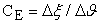(5)

Equation (5) is detailed as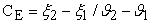(6)

Where Δξ = Change in emitted carbon at two values of TGF ϑ 2, ϑ 1.

Considering the points (1062, 164.61) & (6389.02, 990.3), (1062, 167.67) & (6389.02, 988.74) and (1062,163.88) & (6389.02, 990.08) as shown in Figure 8, then designating them as (ζ1, ϑ1) & (ζ2, ϑ2) for experimental, derived model and regression model predicted results respectively, and also substituting them into equation (6), gives the slopes: 0.155, 0.154 and 0.155 Tonnes/ Mscfd-1 as their respective emitted carbon per unit TGF.

3.5. Deviational Analysis

Critical analysis of the emitted carbon obtained from experiment and derived model show low deviations on the part of the model-predicted values relative to values obtained from the experiment. This was attributed to the fact that the flow properties of the flared gases and the physico-chemical interactions between the flared gases and the emitted carbon which played vital roles during the flaring process were not considered during the model formulation. This necessitated the introduction of correction factor, to bring the model-predicted emitted carbon to those of the corresponding experimental values.

The deviation Dv, of model-predicted emitted carbon from the corresponding experimental result is given by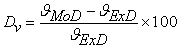(7)

Where ϑExD and ϑMoD are TGF obtained from experiment and derived model respectively.

Figure 9. Variation of model-predicted quantity of emitted carbon (relative to TGP) with associated deviation from experiment

Deviational analysis of Figure 9 and Figure 10 indicate that the precise maximum deviation of model-predicted emitted carbon from the experimental results is 2.71%. This invariably translated into over 97% operational confidence for the derived model as well as over 0.97 reliability response coefficients of emitted carbon to TGP and TGF.

Consideration of equation (7) and critical analysis of Figure 9 and Figure 10 show that the least and highest magnitudes of deviation of the model-predicted emitted carbon (from the corresponding experimental values) are – 0.16 and - 2.71%. Figure 9 and Figure 10 indicate that these deviations correspond to emitted carbon: 988.74 and 459.18 Tonnes, TGP: 9009.3 and 3745 Mscf/d, as well as TGF: 6389.02 and 3045 Mscf/d respectively.

Correction factor, Cf to the model-predicted results is given by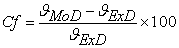(8)

Critical analysis of Figure 9, Figure 10 and Table 5 indicates that the evaluated correction factors are negative of the deviation as shown in equations (7) and (8).

Figure 10. Variation of model-predicted quantity of emitted carbon (relative to TGF) with associated deviation from experiment

#### Table 5. Variation of correction factor (to model-predicted emitted carbon) with TGP and TGF

Table 5 shows that the least and highest correction factor (to the model-predicted emitted carbon) are + 0.16 and + 2.71%. Since correction factor is the negative of deviation as shown in equations (7) and (8), Table 5, Figure 9 and Figure 10 indicate that these highlighted correction factors corresponds to to emitted carbon: 988.74 and 459.18 Tonnes, TGP: 9009.3 and 3745 Mscf/d, as well as TGF: 6389.02 and 3045 Mscf/d respectively.

The correction factor took care of the negligence of operational contributions of the flow properties of the flared gases and the physico-chemical interactions between the flared gases and the emitted carbon which actually played vital role during the flaring process. The model predicted results deviated from those of the experiment because these contributions were not considered during the model formulation. Introduction of the corresponding values of Cf from equation (8) into the model gives exactly the corresponding experimental values of emitted carbon.

It is very pertinent to state that the deviation of model predicted results from that of the experiment is just the magnitude of the value. The associated sign preceding the value signifies that the deviation is a deficit (negative sign) or surplus (positive sign).

### 4. Conclusion

Following a simulative analysis of emitted carbon (during flaring process) based on total gas produced and total gas flared, quantity of emitted gas increases with increase in both total gas produced (TGP) and total gas flared (TGF). A two-factorial model, derived and validated was used for the empirical analysis. The emitted carbon is a linear function of TGP and TGF. The validity of the derived model expressed as: was rooted in the model core expression ξ - 30.7738 = 0.0513 ₰ + 0.0776 ϑ where both sides of the expression are correspondingly approximately equal. Results from evaluations indicated that the standard error incurred in predicting emitted carbon for each value of the TGP & TGF considered, as obtained from experiment, derived model and regression model were 14.2963, 7.4141 and 14.823 & 1.3657, 7.4084 and 0.0039 % respectively. Further evaluation indicates that emitted carbon per unit TGF as obtained from experiment, derived model and regression model were 0.155, 0.154 and 0.155 Tonnes/Mscfd-1 respectively. Comparative analysis of the correlations between emitted carbon and TGP & TGF as obtained from experiment, derived model and regression model were all > 0.99. The maximum deviation of the model-predicted emitted carbon (from experimental results) was less than 3%. This translated into over 97% operational confidence for the derived model as well as over 0.97 reliability response coefficients of emitted carbon to TGP and TGF.

### References

  Onyejekwe, I. M. (2012). Health Impact Analyses of Gas Flaring in the Niger Delta. IREJEST, 9 (1): 94-100.In article  Abraham, A, and Baikunth N., (2000). “Hybrid Intelligent Systems: A Review of a decade of Research”, School of Computing and Information Technology, Faculty of Information Technology, Monash University, Australia, Technical Report Series, 5 (2000): 1-55.In article  Berenji, H. R. and Khedkar, P. (1992). “Learning and Tuning Fuzzy Logic Controllers through Reinforcements”, IEEE Transactions on Neural Networks, 3: 724-740.In article CrossRef PubMed  Czogala, E. and Leski, J. (2000). “Neuro-Fuzzy Intelligent Systems, Studies in Fuzziness and Soft Computing”, Springer Verlag, Germany.In article CrossRef  Ezzati, M. and Kammen, D. M. (2002). Household Energy, Indoor Air Pollution and Health in Developing Countries. Knowledge Base for Effective Interventions. pp 12.In article  Hewitt, D. N., Sturges, W. T., and Noa, A. (1995).Global Atmospheric Chemical Changes, New York. Chapman and Hall. pp 56.In article  Botkin, D. B. and Kella, E. A. (1998): Environmental Science, Earth as living Planet. Second Edition. John Wiley and Sons, Canada. pp 123.In article  Kindzierski, W. D. (2000). Human Environment Exposure to Hazardous Air Pollutants from Gas Flare. Environmental Reviews. 8: 41-62.In article CrossRef  Strosher, M. (1996).Investigation of Flare Gas Emissions in Alberta, Canada: Alberta Research Council.In article  Manby, B., (1999). The Price of Oil: Corporate Responsibilty and Human Rights Violations in Nigerians Oil Producing Communities. Human Right Watch New York. pp. 202.In article  Selective catalytic reduction, (SCR) De-NOx Technologies, http://www.de-nox-com/SCR.htmlIn article  Selective non-catalytic reduction (SNCR), De-NOx Technologies, http://www.de-nox.com/technology.htmIn article  Sulfur Dioxide Scrubbers, Duke Energy, http://www.duke-energy.com/environment/air-quality/sulphur-dioxide-scrubbers.aspIn article  Nwoye, C. I. (2008). Data Analytical Memory; C-NIKBRAN.In article PubMed